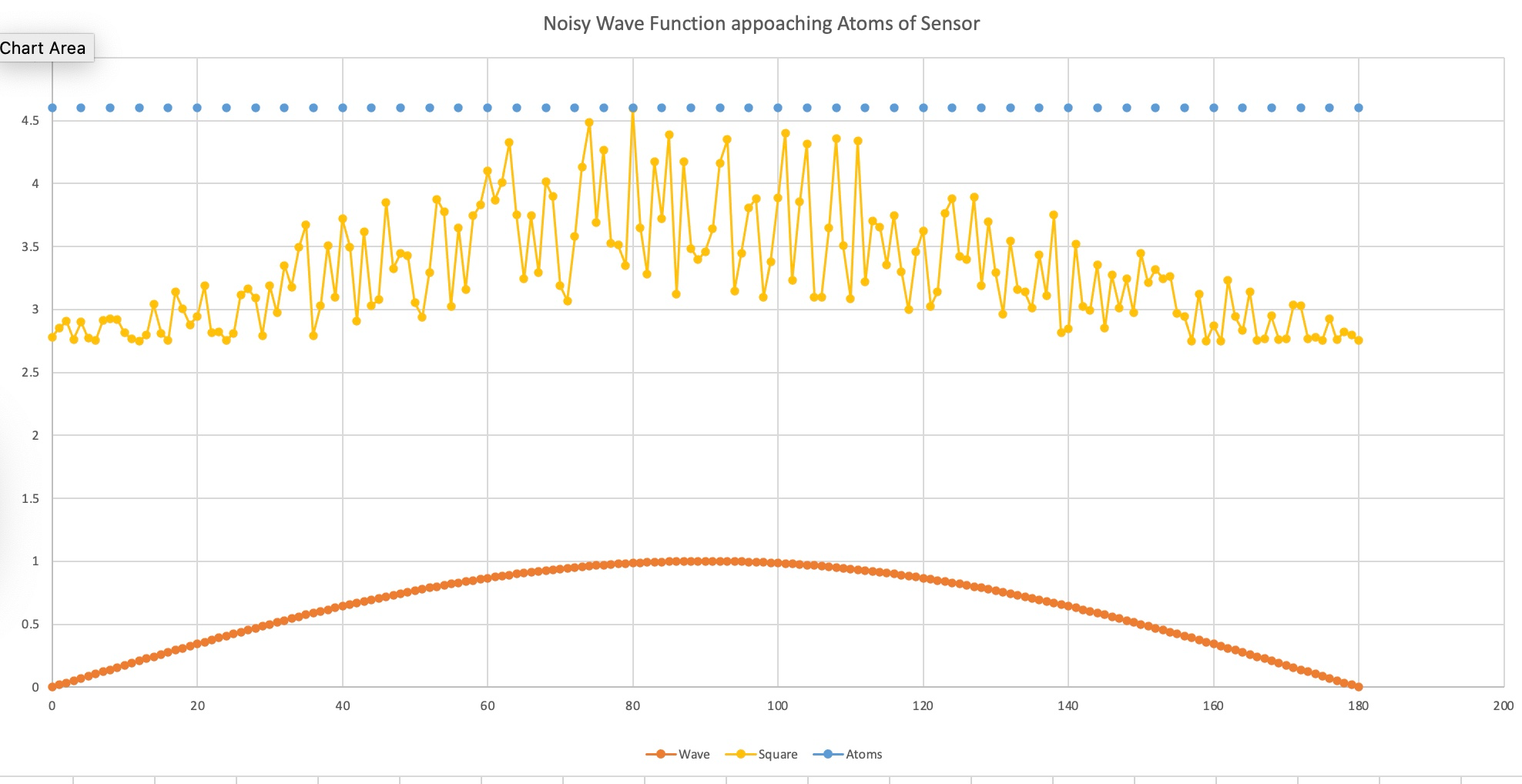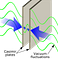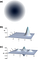# Measuring the Quantum FoamFigure 1, The smooth curve is the electron wave amplitude, and in yellow above, combined with the quantum noise, approaching the phosphor atoms. The noise peak at 80 degrees wins in this example, and all the electron energy will be absorbed by that atom.

Introduction

Quantum foam or spacetime foam is the quantum fluctuation of spacetime on very small scales due to quantum mechanics. The idea was devised by John Wheeler in 1955.

Very little is known about the quantum foam or fluctuations, or vacuum energy. We don’t even have a decent name for it. It is thought that it is related to the source of dark energy, and other important phenomena in the universe. Many physicists think that learning about that will bring about the next big advances in physics. In this article, I will show you three ways to measure it. They are:

1. Measuring the statistics of of the electron or photon after scattering through an aperture and absorption by atoms to measure the noise in the quantum foam.
2. The Casimir effect measurements
3. Quantum gravity measurements

Electron or Photon Scattering Measurements

Let us consider an electron in a cathode ray tube being guided in its path by electric and magnetic fields to a phosphor that lights up upon absorbing that electron. What is really happening? From quantum mechanics, the electron in free space is a wave, and it diffracts from edges, and interferes with other electron waves. The double slit experiment illustrates this wonderfully.

As the electron wave approaches the atoms in the phosphor, there are many choices for which atom the electron wave will choose to mate with. The question of how it is decided which atom is chosen is the source of many controversies in the interpretation of the meaning of quantum mechanics.

Here is my model of the universe and the electron. The electron wave is a pure coherent wave. This wave has no noise of its own, but it is propagating in the noisy environment of the quantum foam that fills the universe. There is so much energy in the quantum foam that there is always a chance that an electron-positron pair will spontaneously appear anywhere in time and space. That is due to the quantum fluctuations of free space have a certain probability of locally and briefly exceeding the threshold with enough energy (1.022 Mev) to create those two particles. Vacuum fluctuations appear as virtual particles, which are always created in particle-antiparticle pairs. Since they are created spontaneously without a source of energy, vacuum fluctuations and virtual particles are said to violate the conservation of energy. I mention that because it means that there is enough energy in the quantum vacuum to significantly add noise to the coherent electron to distort the electron wave.

Figure 1 shows a model of how the coherent electron wave adds to the random quantum field to make a very noisy electron wave. This wave can reach any of many atoms in the phosphor, and ultimately it is absorbed by one of them. The quantum foam, or noise as I call it, causes fluctuations that can result in absorption by an atom that is not directly in line with the average path of the electron wave. This is why they call it a probability wave. That is wrong. It is an electron wave. It has noise added to it by the quantum foam that gives it that probability character.

There is no need for multiple universes, or hidden variables, or any of the many interpretations of quantum mechanics. It is simply noise in the quantum foam. When the matter wave of the electron gets close enough to the selected atom, randomly due to the noise, entanglement causes all the energy of the original electron to combine with the absorbing atom, and puts the atom into a new state that makes the atom emit light. How fast this absorption of the electron wave happens, I don’t know. It could be faster than the speed of light, or at the speed of light or less than the speed of light. This is something for experimentalists to explore.

Measurements

So, what experiments can be done to learn more about the quantum foam? First we can measure the statistics of the absorption of electrons by phosphors after many runs of the experiment. I think the easiest way would be to do the single slit experiment, but many other configurations could be used.

A long slit of infinitesimal width which is illuminated by light diffracts the light into a series of circular waves and the wavefront which emerges from the slit is a cylindrical wave of uniform intensity, in accordance with Huygens–Fresnel principle. Any statistical deviation from this pattern of interaction probabilities would tell us more about the quantum foam.

We know almost nothing about the quantum foam and this should tell us something new. We don’t know the characteristics of the noise. It could be white noise (uniform amplitude at all frequencies), or 1/f noise ( the noise amplitude decreases by 1/f). We also don’t know the type of statistics the noise follows. Some different statistics that are known about are: Bose-Einstein, Fermi-Dirac, and Maxwell-Boltzmann. All of these types of noise are called Gaussian noise. There is also non-Gaussian noise. This will be explained more below.

Bose–Einstein distribution statistics apply when an unlimited number of bosons can condense into the same energy state. In condensed matter physics, a Bose–Einstein condensate (BEC) is a state of matter (also called the fifth state of matter) which is typically formed when a gas of bosons at low densities is cooled to temperatures very close to absolute zero(-273.15 °C, -459.67 °F). Under such conditions, a large fraction of bosons occupy the lowest quantum state, at which point microscopic quantum mechanicalphenomena, particularly wavefunction interference, become apparent macroscopically.

This distribution could be true for photons, but not for electrons. We have immediately discovered that electrons and photons could have different statistics. So, the measurements will need to be done for both.

Fermi–Dirac distribution statistics apply to fermions (particles that obey the Pauli exclusion principle) as electrons do.

Maxwell-Boltzmann statistics apply at high temperatures and are the classical limit where we live.

So, we can make a guess that Bose_Einstein will apply to photons, and Fermi-Dirac will apply to electrons. But, that is only a guess. Both fermions, and bosons are created out of the quantum foam, so we don’t really know what will happen.

I want to make a side note here that there are many different types of waves in the universe. We don’t really know how many. In general, we loosely refer to them as waves, and wave functions as if they are all the same. I think that is a problem. We need to get specific. For the electron, it has an electromagnetic field surrounding it, but the mass is made of waves in the Higgs field. The photon is made of waves in the electromagnetic field. The quarks also are waves in the Higgs field. There are also gravitational waves as well, and I don’t know which field that is manifesting. (Higgs?)

Casimir Effect Measurements

Other measurements that can and are being made are experiments on the Casimir force.Figure 3: Illustration of Casimir forces between parallel plates. source: Marco Tavaro Ph.d. in Medium

This force is caused by a difference between the force on the outside of two parallel metal plates, and the inside of these plates as shown in Figure 3. The force on the outside of the plates is greater than the force on the inside because the vacuum electromagnetic fluctuations on the outside of the plates are greater than the inside. On the inside, the low frequency waves are excluded because, with the small distance, the wavelength cannot be greater than that distance. This force is very small and hard to measure. The plates must be 100 nm or less apart for the force to be large enough to be measurable. But, it is measuring the ripples in the quantum field. The theory is that the waves that the Casimir measurements are measuring are electromagnetic waves. I wonder about that. If that is true, the Casimir force should go to zero if one shields the plates in a Faraday cage, which blocks all electromagnetic radiation. I don’t know if they have tried that experiment. If the force does not go away, it must be caused by a different wave. The Higgs field would be one alternative, because the Higgs field cannot be blocked.

Because it is very difficult to hold two plates of large size parallel to better than about 10 nm that would be required for a decent measurement, researchers use one flat plate and one spherical plate.

To measure such small forces, new methods have had to be developed such as that in Figure 2. The force measured in this experiment is in the hundreds of pico-newtons. These measurements should be done in different environments such as with and without the Faraday cage.

Quantum gravity measurements

Some researchers in quantum computation have also discovered another way to measure the characteristics of the quantum foam. The paper describing this proposal is:

Richard Howl et al, Non-Gaussianity as a Signature of a Quantum Theory of Gravity, PRX Quantum 2, 010325 — Published 17 February 2021, https://journals.aps.org/prxquantum/pdf/10.1103/PRXQuantum.2.010325

The authors have used recent findings in the field of quantum information science to develop a new approach to determining how our Universe works at its most fundamental level. For more than a hundred years, physicists have struggled to understand how the two foundational theories of science, quantum theory and general relativity, which respectively describe microscopic and macroscopic phenomena, are unified into a single overarching theory of nature. During this time, two fundamentally contrasting approaches have been developed, called ‘quantum gravity’ QG and ‘classical gravity’ CG. However, a complete lack of experimental evidence means that we do not know which approach the overarching theory actually takes.

Now, a collaboration between researchers in quantum information, quantum gravity, and quantum technology, demonstrates that only quantum and not classical gravity could bring a signature (called non-Gaussianity) that are typically discussed in the context of quantum computation, connecting the fields of quantum gravity, quantum information, and computing.Illustration of the proposal for a tabletop test of QG. (a) A single spherical BEC of 109 atoms and radius R = 200 μm is left to self-interact gravitationally for around t = 2 s. If QG acts, non-Gaussianity is induced in its quantum state, whereas if CG acts, Gaussianity is preserved. Each atom is equally delocalized across the extent of the BEC but, since the BEC is in a spherical harmonic trap, the density of the BEC is greatest at its center and drops off to zero asymptomatically, as illustrated.

The BEC is initially in a Gaussian state, and its Wigner function W is displayed in (b). Here, for simplicity, a coherent state |α⟩ isassumed, but this can also be squeezed to increase the signal-to-noise ratio, as discussed in the main text. If QG acts, and the interaction time were long enough, then gravity could even force √ the coherent state to a Yurke-Stoler cat state (|α⟩ + i| − α⟩)/ 2. The Wigner function for such a non-Gaussian state is illustrated in ©. If, however, CG acts, then the state will remain Gaussian. In the nonrelativistic CG limit, the state will remain a coherent state, whereas relativistic CG effects would, in principle, squeeze the state but keep it Gaussian. In practice, the interaction time would not be long enough for such a dramatic effect as a coher- ent state changing to a Yurke-Stoler state, and instead smaller deviations from a Gaussian distribution are sought (see the main text). α = 2 is used in the plots, whereas in practice the BEC will have an amplitude of around |α| = 104.5, the square root of the number of atoms.

This is the way they propose a way to test experimentally the quantum character of gravity in a scientific laboratory: billions of atoms are cooled to very low temperatures in a spherical trap such that they enter a new phase of matter, called a Bose-Einstein condensate, and act like a single ‘wave of matter’ inside the trap. This single wave is massive enough to feel its own gravitational pull, and if after a certain period of time it demonstrates non-Gaussianity, then this proves that nature must take the quantum gravity approach. The proposed experiment involves just a single quantum system and does not rely on assumptions concerning the locality of the interaction, making it simpler than previous approaches and potentially expediting the delivery of the first experimental test of quantum gravity. After more than a hundred years of research, having information on the unified theory of nature may be closer. The details of this are beyond the scope of this article, but are given in the reference.

Conclusion

The problem of what is going on at the smallest regime of the universe has been mystifying us forever. The Quantum foam is so poorly understood that we cannot figure out what it is made of. It has fields, waves in the fields, entanglement, possible other dimensions, uncertainty, Gaussian statistics, Non-Gaussian statistics, and many other mysteries. We need measurements. This article is intended to point out some of the measurements that need to be made for us to make progress. This is definitely not all encompassing. Many more opportunities for measurement surely must exist. We will figure this out.

Let’s try to determine what the measurements that are proposed here might find out.

1. Measuring the statistics of of the electron or photon after scattering through an aperture to measure the noise in the quantum foam. This will allow us to find out several things. First, what are the statistics of how the quantum foam affects the trajectory of the electron/photon with different measurement conditions with or without a Faraday cage, and other variations. We need to find out if the statistics are Gaussian or non-Gaussian. This would be a big deal, because if the statistics are non- Gaussian, it would be big breakthrough in Quantum gravity.
2. The Casimir force has been known about for many years, but not much progress has been made in deriving much information about the quantum foam. A key analysis of this phenomena is that free space is filled with waves. The low frequency waves cannot be maintained in between very closely spaced electrodes. Therefore, the force between the metal plates is less than the force on the outside. I don’t know what useful information about the quantum foam we can get from that.
3. Quantum gravity measurements are needed to determine the true nature of gravity. This may seem off topic, but gravity is an intimate part of the quantum foam. The theoretical link between quantum gravity and quantum information, and that quantum information theory requires that quantum gravity to be non-Gaussian is a breakthrough. It will make the measurements of the statistics of the behavior of electrons have more meaning.

There may be many other methods of measuring the quantum foam, but these are all I can find so far.

Postscript: If there are any errors or misconceptions in this article, please let me know. I don’t have anyone that knows anything about quantum mechanics to run this by. So, you are it.

I am a retired industrial physicist interested in the fundamental physics of the universe.

## More from John Karpinsky

I am a retired industrial physicist interested in the fundamental physics of the universe.